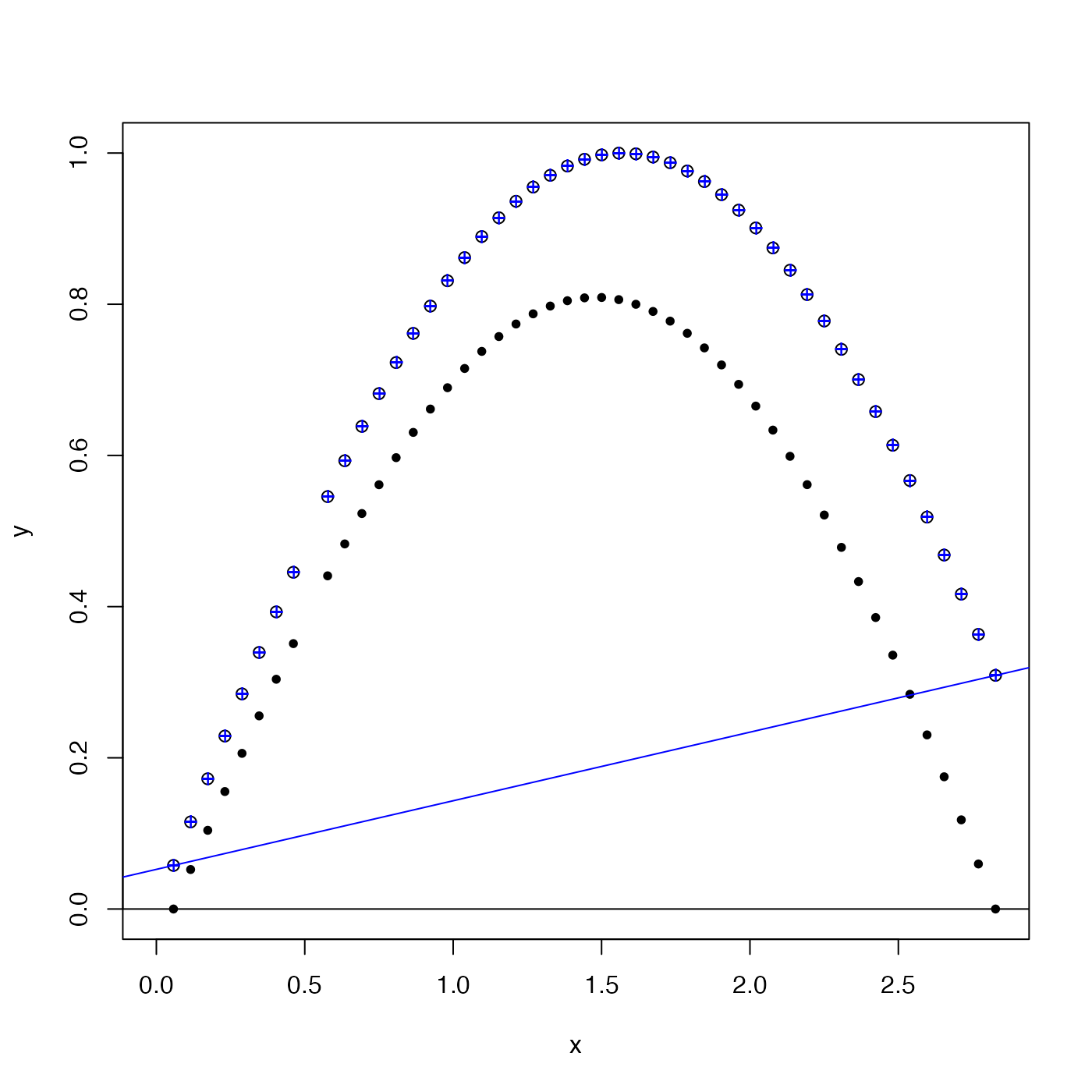Detrends y by subtracting a linear trend in x, to create a vector that is zero for its first and last finite value. If the second parameter (y) is missing, then x is taken to be y, and a new x is constructed with seq_along(). Any NA values are left as-is.

detrend(x, y)

## Arguments

x a vector of numerical values. If y is not given, then x is taken for y. an optional vector

## Value

A list containing Y, the detrended version of y, and the intercept a and slope b of the linear function of x that is subtracted from y to yield Y.

## Details

A common application is to bring the end points of a time series down to zero, prior to applying a digital filter. (See examples.)

## Examples

x <- seq(0, 0.9 * pi, length.out=50)
y <- sin(x)
y <- NA
y <- NA
plot(x, y, ylim=c(0, 1))d <- detrend(x, y)
points(x, d$Y, pch=20)abline(d$a, d$b, col='blue')abline(h=0)points(x, d$Y + d$a + d$b * x, col='blue', pch='+')